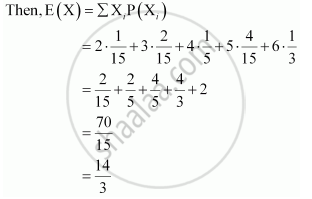Share

# Two Numbers Are Selected at Random (Without Replacement) from the First Six Positive Integers. Let X Denotes the Larger of the Two Numbers Obtained. Find E(X). - CBSE (Commerce) Class 12 - Mathematics

ConceptRandom Variables and Its Probability Distributions

#### Question

Two numbers are selected at random (without replacement) from the first six positive integers. Let X denotes the larger of the two numbers obtained. Find E(X).

#### Solution

The two positive integers can be selected from the first six positive integers without replacement in 6 × 5 = 30 ways

X represents the larger of the two numbers obtained. Therefore, X can take the value of 2, 3, 4, 5, or 6.

For X = 2, the possible observations are (1, 2) and (2, 1).

:. P(X=2) = 2/30 = 1/15

For X = 3, the possible observations are (1, 3), (2, 3), (3, 1), and (3, 2).

:. P(X=3) = 4/30 = 2/15

For X = 4, the possible observations are (1, 4), (2, 4), (3, 4), (4, 3), (4, 2), and (4, 1).

:. P(X=4) = 6/30 = 1/5

For X = 5, the possible observations are (1, 5), (2, 5), (3, 5), (4, 5), (5, 4), (5, 3), (5, 2), and (5, 1).

:. P(X=5) = 8/30 = 4/15

For X = 6, the possible observations are (1, 6), (2, 6), (3, 6), (4, 6), (5, 6), (6,5) , (6, 4), (6, 3), (6, 2), and (6, 1).

:. P(X=6) = 10/30 = 1/3

Therefore, the required probability distribution is as follows.

 X 2 3 4 5 6 P(X) 1/15 2/15 1/5 4/15 1/3Is there an error in this question or solution?

#### Video TutorialsVIEW ALL 

Solution Two Numbers Are Selected at Random (Without Replacement) from the First Six Positive Integers. Let X Denotes the Larger of the Two Numbers Obtained. Find E(X). Concept: Random Variables and Its Probability Distributions.
S